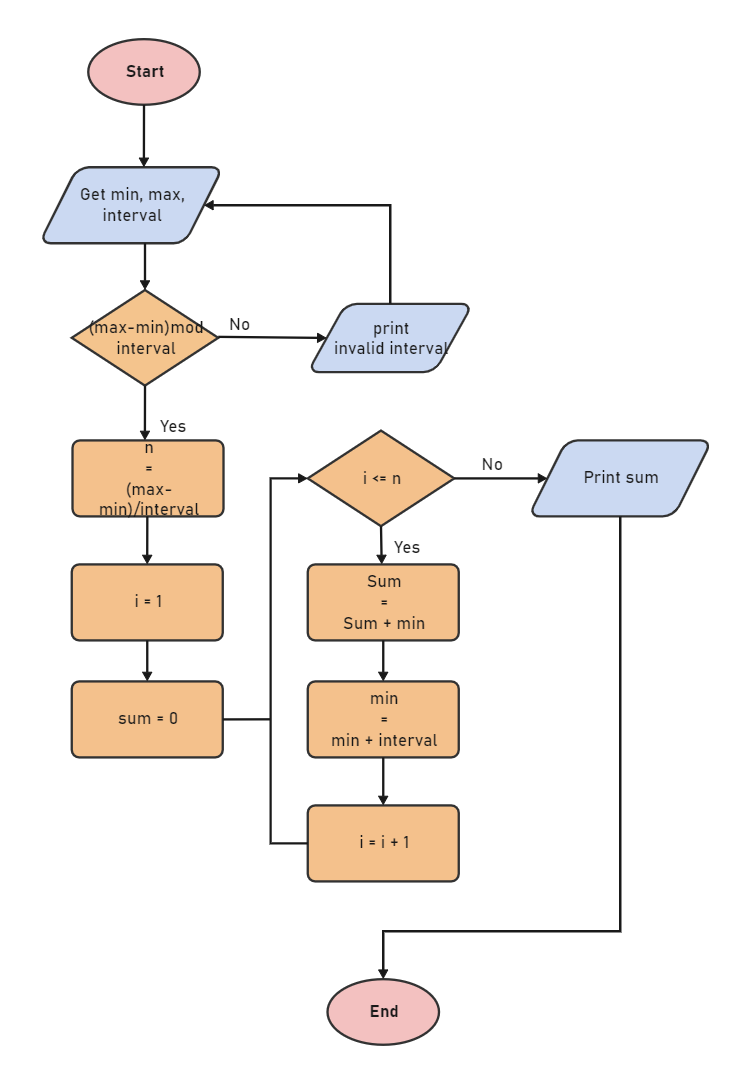Template Community / Mathematics Algorithm Flowchart

# Mathematics Algorithm FlowchartCharlotte
Published on 2021-07-16
Edit OnlineFlowchart for an algorithm that gets two numbers and prints the sum of their value and odd numbers in a given interval and their sum and count, start. The process starts by entering the values of min, maximum, and interval. The simple mathematics algorithm diagram shows how integers are assigned a particular value, and if that value is less than equal to the n, then the print sum command would work. If sum = sum + min, then the System will assign the value of min + interval to the min and increment integer with plus one. Use EdrawMax to create mathematics algorithm diagrams for your academics project.
Tag
science diagram
Mathematics Diagram
Report
1
389PostRecommended Templates